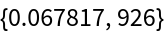#Function Repository Resource:

# MaximalSubsets

Prune non-maximal subsets from a list of subsets

Contributed by: Carl Woll
 ResourceFunction["MaximalSubsets"][list] prunes non-maximal subsets from the sets in list.

## Details and Options

The argument list should be a list of subsets, where each subset is a list of elements.
A non-maximal subset is a list which is completely contained in another subset.

## Examples

### Basic Examples

Here is a list of sets:

 In:=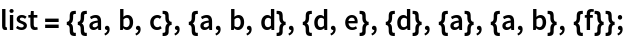Remove the non-maximal subsets {d}, {a}, and {a,b}:

 In:=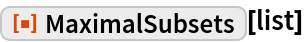Out=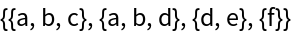### Scope

MaximalSubsets can handle a large list of subsets:

 In:=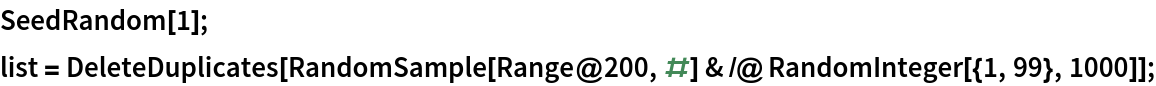The number of maximal subsets:

 In:=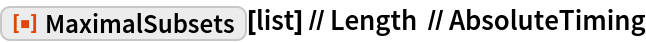Out=